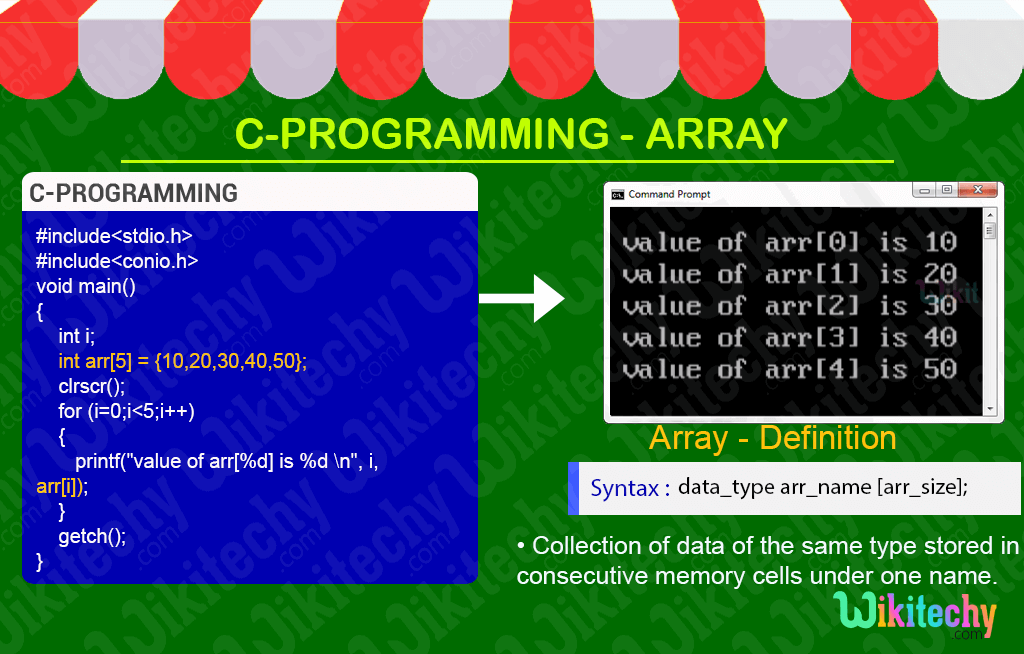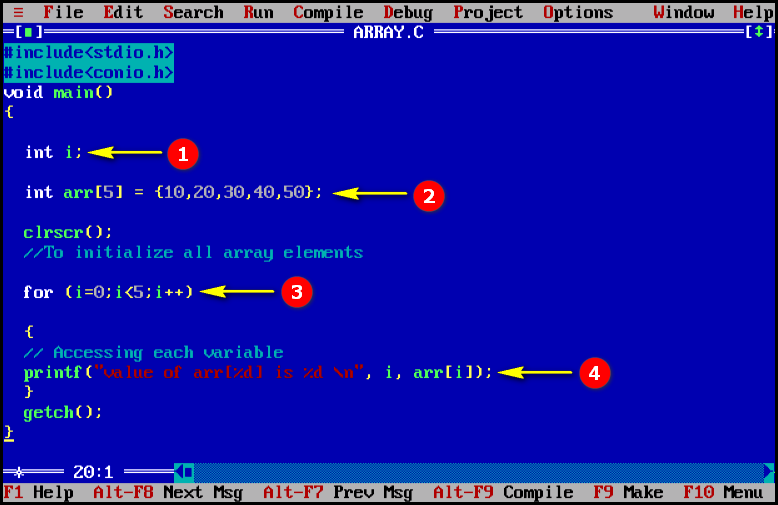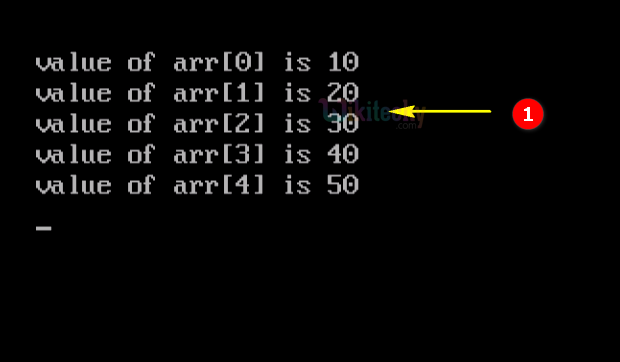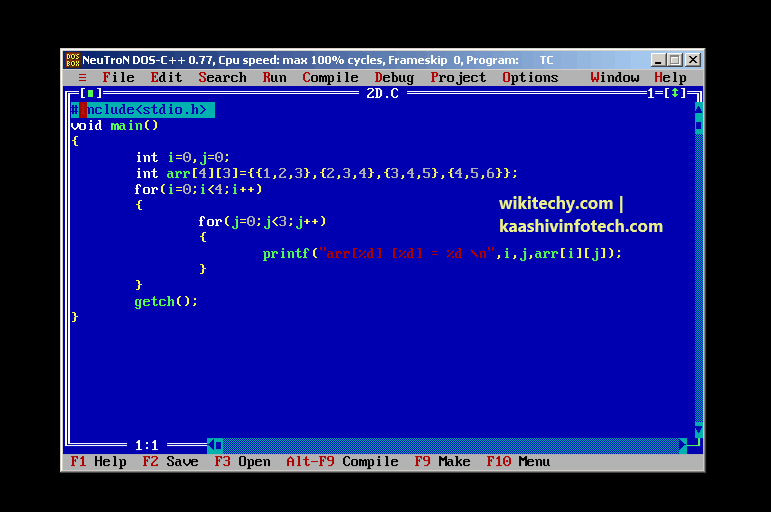# Array in C

## Arrays and Strings in TamilLearn C - C tutorial - Array in c - C examples - C programs

## C Array - Definition and Usage

• In C- Programming Array is a data structure, which is used for storing the collection of data of same type under single variable name.
• Basically the array values are defined as constant.
• Array variable names contains only letter, digit and underscore characters.• Array names cannot begin with a digit character. I.e., The rule for giving the array name is same as the ordinary variable.
• There are 2 types of C arrays. They are,
1. One dimensional array
2. Multi-dimensional array
• Two Dimensional Array,
• Three Dimensional Array,
• Four Dimensional Array … etc…

## Advantages of Arrays in C

• Code Optimization : Less code to the access the data.
• Easy to traverse data : By using the for loop, we can retrieve the elements of an array easily.
• Easy to sort data : To sort the elements of array, we need a few lines of code only.
• Random Access : We can access any element randomly using the array.## Disadvantages of Arrays in C

• Fixed Size: Whatever size, we define at the time of declaration of array, we can't exceed the limit. So, it doesn't grow the size dynamically like Linked List.
• Insert/delete records: Inserting and deleting the records from the array would be costly since we add / delete the elements from the array, we need to manage memory space too.## C Syntax## Initialization of Array in C

• A simple way to initialize array is by index. Notice that array index starts from 0 and ends with [SIZE - 1].## C Code - Explanation1. Here in this statement we declare and assign the variable “i” as integer.
2. In this statement we declare the variable “arr” and initialize the value of the variable as integer.
3. In this statement we execute the for loop.
4. Here in this printf statement we print the value of the array variable.

## Sample Output - Programming Examples1. Here in this output the array index with its array values are displayed in this console window of C.

## One - dimensional Arrays in C

• A one-dimensional array or a single dimension array is a type of linear array.
• Accessing its elements involves a single subscript which can either represent a row or column index.## Two Dimentional Array

• The two dimensional array in C language is represented in the form of rows and columns, also known as matrix.
• It is also known as array of arrays or list of arrays. The two dimensional, three dimensional  or other dimensional arrays are also known as multidimensional arrays.## Syntax• A way to initialize the two dimensional array at the time of declaration is given below:
`int arr={{1,2,3},{2,3,4},{3,4,5},{4,5,6}};`

## Sample Code: